Next: Normal Reflection and Transmission Up: Traveling Waves Previous: Energy Conservation

# Transmission Lines

A transmission line is typically used to carry high frequency electromagnetic signals over long distances: that is, distances sufficiently large that the phase of the signal varies significantly along the line (which implies that the line is much longer than the wavelength of the signal). A common example of a transmission line is an ethernet cable.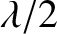In its simplest form, a transmission line consists of two parallel conductors that carry equal and opposite electrical currents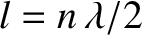, where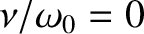measures distance along the line. (See Figure 37.) Let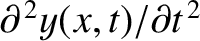be the instantaneous voltage difference between the two conductors at position. Consider a small section of the line lying betweenand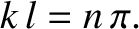. If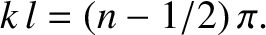is the electric charge on one of the conducting sections, and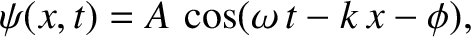the charge on the other, then charge conservation implies that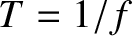. However, according to standard electrical circuit theory (Fitzpatrick 2008),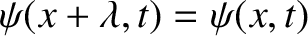, where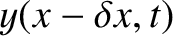is the capacitance per unit length of the line. Standard circuit theory also yields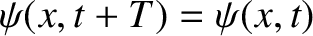(ibid.), where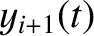is the inductance per unit length of the line. Taking the limit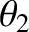, we obtain the so-called Telegrapher's equations (ibid.),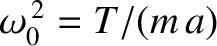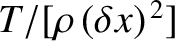(412)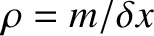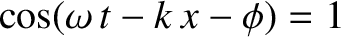(413)

(See Exercise 7.) These two equations can be combined to give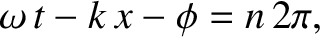(414)

together with an analogous equation for. In other words,andboth obey a wave equation of the form (383) in which the associated phase velocity is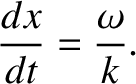(415)

Multiplying (412) by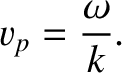, (413) by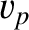, and then adding the two resulting expressions, we obtain the energy conservation equation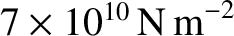(416)

where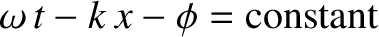(417)

is the electromagnetic energy density (i.e., energy per unit length) of the line, and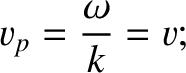(418)

is the electromagnetic energy flux along the line (i.e., the energy per unit time that passes a given point) in the positive-direction (ibid.). Consider a signal propagating along the line, in the positive-direction, whose associated current takes the form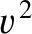(419)

where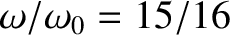andare related according to the dispersion relation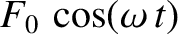(420)

It can be demonstrated, from Equation (412), that the corresponding voltage is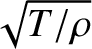(421)

where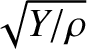(422)

Here,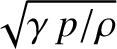(423)

is the characteristic impedance of the line, and has units of ohms. It follows that the mean energy flux associated with the signal is written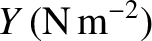(424)

Likewise, for a signal propagating along the line in the negative-direction,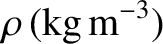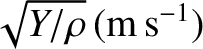(425)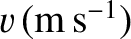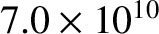(426)

and the mean energy flux is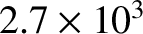(427)

As a specific example, consider a transmission line consisting of two uniform parallel conducting strips of width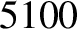and perpendicular distance apart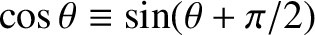, where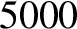. It can be demonstrated, using standard electrostatic theory (Grant and Philips 1975), that the capacitance per unit length of the line is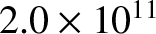(428)

whereis the electric permittivity of free space. Likewise, according to standard magnetostatic theory (ibid.), the line's inductance per unit length takes the form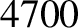(429)

where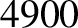is the magnetic permeability of free space. Thus, the phase velocity of a signal propagating down the line is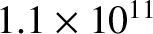(430)

which, of course, is the velocity of light in vacuum. [See Equation (479).] This is not a coincidence. In fact, it can be demonstrated that the inductance per unit length and the capacitance per unit length of any (vacuum filled) transmission line satisfy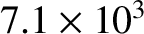(431)

Hence, signals always propagate down such lines at the velocity of light in vacuum. The impedance of a parallel strip transmission line is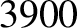(432)

where the quantity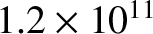(433)

is known as the impedance of free space. However, because we have assumed that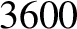, it follows that the impedance of the line is impractically high (i.e.,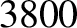).

Practical transmission lines generally consist of two parallel wires twisted about one another (for example, twisted-pair ethernet cables), or two concentric cylindrical conductors (for example, co-axial TV cables.) For the case of two parallel wires of radius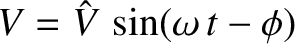and distance apart(where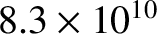), the capacitance per unit length is (Wikipedia contributors 2012)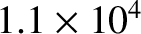(434)

Thus, the impedance of a parallel wire transmission line becomes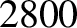(435)

For the case of a co-axial cable in which the radii of the inner and outer conductors areand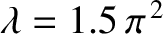, respectively, the capacitance per unit length is (Fitzpatrick 2008)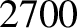(436)

Thus, the impedance of a co-axial transmission line becomes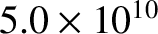(437)

It follows that a parallel wire transmission line of given impedance can be fabricated by choosing the appropriate ratio of the wire spacing to the wire radius. Likewise, a co-axial transmission line of given impedance can be fabricated by choosing the appropriate ratio of the radii of the outer and inner conductors.Next: Normal Reflection and Transmission Up: Traveling Waves Previous: Energy Conservation
Richard Fitzpatrick 2013-04-08